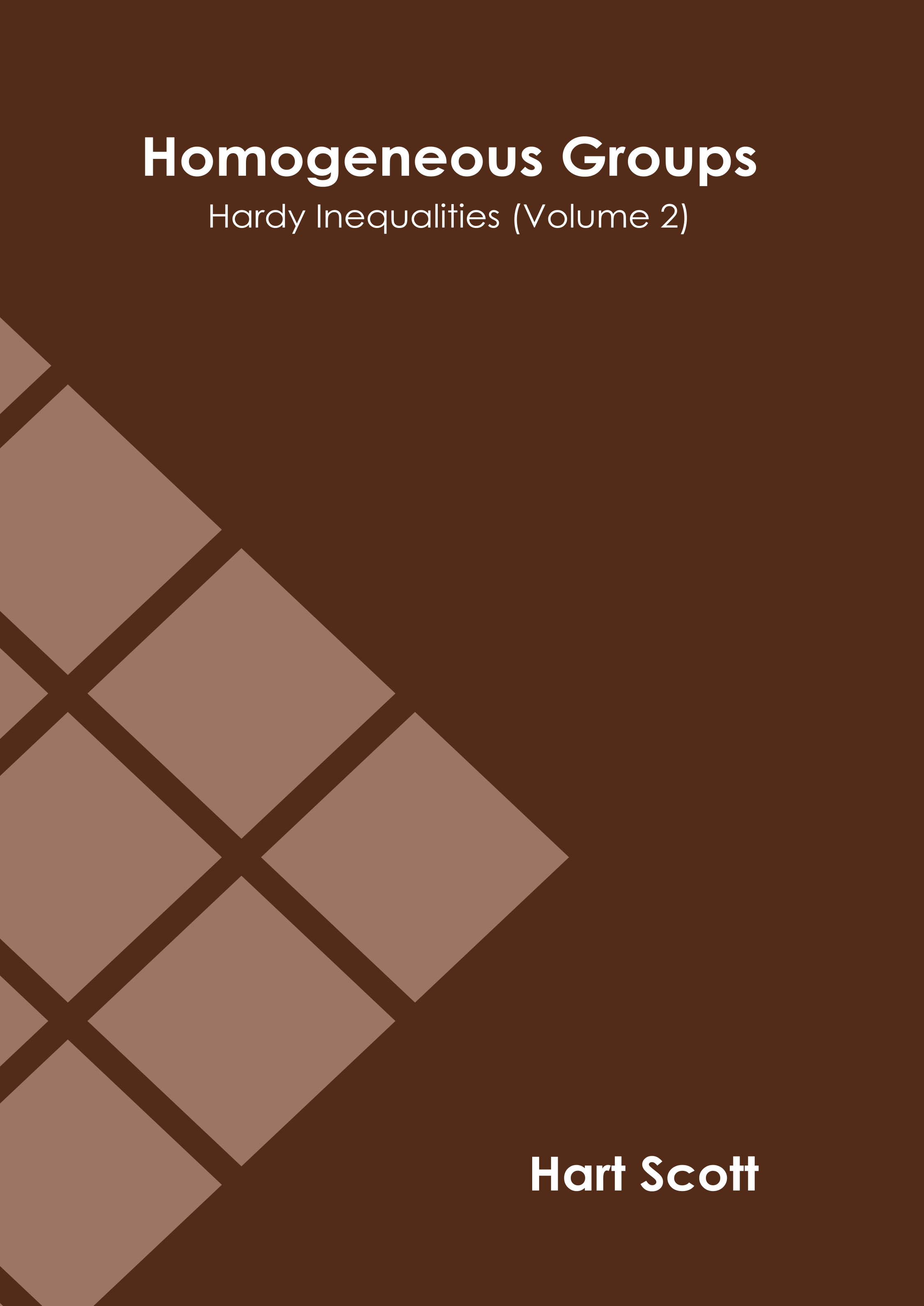• library@msu.ac.th
• Academic Resource Center Mahasarakham University
• library@msu.ac.th
• Academic Resource Center Mahasarakham Universityหมวดหมู่### Homogeneous Groups: Hardy Inequalities (Volume 2)

Homogenous groups are part of the theories of Lie groups, algebraic groups and topological groups. A homogeneous space for a group G is a non-empty manifold or topological space X on which G acts transitively. The elements of G are known as the symmetries of X. When the group G in question is the automorphism group of the space X, a special case arises. An isometry group, diffeomorphism group or a homeomorphism group can be called an automorphism group. In this case, X is homogeneous if naturally X looks locally identical at each point, either in the sense of isometry, diffeomorphism or homeomorphism. Thus there is a group action of G on X which can be thought of as preserving some geometric structure on X, and making X into a single G-orbit. This book outlines the processes and applications of homogenous groups in detail. It presents this complex subject in the most comprehensible and easy to understand language. This textbook will serve as a valuable source of reference for graduate and post graduate students.

ใส่ตะกร้า
• ISBN9781639873081
• ประเภท E-Book
• ผู้แต่ง Hart Scott
• สำนักพิมพ์ Murphy & Moore Publishing
• ครั้งที่พิมพ์ 1
• ปีที่พิมพ์2021
• ภาษาภาษาอังกฤษ
• หมวดหมู่วิทยาศาสตร์
###### : ข้อมูลหนังสือ

Homogenous groups are part of the theories of Lie groups, algebraic groups and topological groups. A homogeneous space for a group G is a non-empty manifold or topological space X on which G acts transitively. The elements of G are known as the symmetries of X. When the group G in question is the automorphism group of the space X, a special case arises. An isometry group, diffeomorphism group or a homeomorphism group can be called an automorphism group. In this case, X is homogeneous if naturally X looks locally identical at each point, either in the sense of isometry, diffeomorphism or homeomorphism. Thus there is a group action of G on X which can be thought of as preserving some geometric structure on X, and making X into a single G-orbit. This book outlines the processes and applications of homogenous groups in detail. It presents this complex subject in the most comprehensible and easy to understand language. This textbook will serve as a valuable source of reference for graduate and post graduate students.